lang="en-GB"> Magnetometer Soft Iron and Hard Iron Calibration: Why and How - Atadiat

# Magnetometer Soft Iron and Hard Iron Calibration: Why and How

IMU series:

One way of determining the geographical direction depends on the magnetic field of the Earth. Because of the geomagnetic effect, we know the direction of the north using the compass. The red part of its needle points toward the north, and the other part of the needle towards the south.The image shows that the red section of the compass needle always points towards the north, which forms the south pole of the Earth’s permanent magnet. image source: Panchuk, Karla. “3.4 Earth’s Magnetic Field.” openpress.usask.ca/physicalgeology/chapter/3-4-earths-magnetic-field-2

The poles of the Earth’s magnetic field do not coincide with the geographical poles, and because of the mismatch, we have two terms: geographical pole and magnetic pole.An image showing the difference between the location of the geographic pole and the magnetic pole. Image source: “What Are The Differences Between Geographic Poles And Magnetic Poles Of The Earth?” WorldAtlas, www.worldatlas.com/articles/what-are-the-differences-between-geographic-poles-and-magnetic-poles-of-the-earth.html

The geographical poles are fixed, which are the meeting places of longitude and latitude in the north and south of the globe. The magnetic poles move periodically for physical reasons related to the terrestrial formation that causes the emergence of this geomagnetic field.The picture shows the locations of the changing magnetic north over the years. Image source: North magnetic pole. In Wikipedia. https://en.wikipedia.org/wiki/North_magnetic_pole

## Finding the geographic directions using a Magnetometer

The angle between the geographic pole and the magnetic pole is determined by scientists and called declination, and there are references that give the value of the declination that should be used depending on our location on the surface of the earth. This explains to us the request to turn on the GPS when using the compass on the mobile phone, where the geographical location is determined, and thus the degree of declination is determined, in addition to other information that is received from the GPS satellites and maybe used for direction confirmation.An example of the value of declination to be used between the geographical and magnetic direction of the city of Makkah Al-Mukarramah in the KSA. The website: magnetic-declination.com

The use of a digital magnetometer provides us with measurements of the magnetic field surrounding the sensor, and thus the geomagnetic field. These sensors provide a digital value that expresses the strength of the magnetic field across 3 axes. This is why it is called a three-axis Magnetometer. The value of the magnetic field measured at a point in 3D space is equal to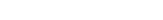We extract the angle degree with a value from 0-360 based on its distance from the north by placing the sensor so that the Z axis is orthogonal with the surface and the magnetic field affects the X and Y axes only. Otherwise, we need to make a tilt-compensated compass which is out of the scoop of this article.The value of the magnetic field at a point in 3-D space using values measured on three axes. Image source: compass heading using magnetometers, Honeywell, Application Note AN-203

Let’s start with a reference case, which is making the X-axis of the sensor parallel to the geomagnetic field so that the value of the field is abblied on one axis only.An illustrative image showing the sensor chip horizontally on a flat surface with the X-axis facing the field towards the north.

We can easily detect the north and south directions when the X-axis is parallel with the field. This is because the value on the X-axis is maximum and positive and on the Y-axis is zero because the geomagnetic field is orthogonal to it.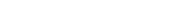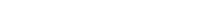In the general case, the sensor is deviated by a certain angle from the field, and for this reason this angle can be calculated, which expresses the value of our distance in degrees from the magnetic north or the geographical north after taking into account the declination between the geographic north and magnetic north based on the geographical location.An illustrative picture of the position of the sensor axes in the general case with the field heading towards the north and forming an angle between the field and the sensor axis.

The angle can be calculated using the well-known trigonometric law: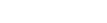Attention must be paid during the practical implementation of the unit of angles and its conversion from radians to degrees and taking into account the angle of declination between the geographical north and the magnetic north.

## Magnetometer Output against 360-degree rotation

The numerical value expressing the field strength ranges from a maximum positive value when the axis is parallel to the field in the same north direction, a zero value when it is perpendicular to it, and a maximum negative value when it is opposite to the north side. It can be expressed in the ideal case as a sinusoidal sign of the X and Y axes when the axis is rotated 360°.The image shows the sensor output in the ideal case on the X and Y axes based on the angle between the field and the axis. It is at the value 0 for the Y-axis, when the X-axis is parallel to the north and Y is orthogonal to the field. Image source: compass heading using magnetometers, Honeywell, Application Note AN-203

We will use a real sensor to assess the output signal of the two axes while rotating the sensor continuously and horizontally between north and south. Below is the shape of the signal using the QMC5883L (GY-271) Compass module.Output signal in uT of the X-axis of QMC5883L sensor with a rotation between east (beginning) , where the axis is perpendicular to the Earth’s magnetic field, to the west passing through the south and then from west to east again (the end) passing through the north. This marks a full rotation of 360 degrees

The shape of the signal is not quite as expected. The first section of the signal that passes from east to west passing through the south has a different maximum value than the second section that passes from west to east passing through the north. By drawing the Y-axis signal, we find that it is worst than the X-axis. It does not reach zero at any angle.Output signal in uT of the Y-axis of QMC5883L sensor with a rotation between east (beginning) ,where the axis is parallel to the Earth’s magnetic field, to the west passing through the south and then from west to east again (the end) passing through the north.This marks a full rotation of 360 degrees

The reason behind this irregular signal shape is due to the presence of nearby magnetic fields that interfere with the geomagnetic field, and this affects the shape of the signal. The other problem that also appears is the mismatch of the direction in which the signal in the X-axis reaches zero in the east or west with the true degree of east and west, in reality, affected by the surrounding side fields. For these reasons, magnetometers need to be calibrated.

## Types of Magnetic Distortion

The first reason for the distortion of the sensed magnetic field is called Hard Iron distortion, and it is caused by the materials which emit a magnetic field, such as magnets, speakers, or motors. This distortion displaces the singal from zero or in other words, it causes an offset.  This type of distortion is easily handled by calculating the offset and subtracting its value from the sensor output.

The second reason for the distortion of the magnetic field is called Soft Iron distortion and its caused by the materials such as iron and nickel which have high magnetic permeability. This type of distortion is more complex and needs complex calculations.

We can express the digital signal of the sensor output more clearly than the sinusoidal signal. This signal should draw in the ideal case a circle with zero centre after calibration, and draw a circle shifted from zero in the case of Hard Iron distortion or the shape of an ellipse in the case of Soft Iron distortion.An illustration of mapping a sinusoidal signal to a circle when moving with angular speed. Image source: “Tracking Simple Harmonic Motion of a Moving Object over Time – dummies.” dummies, 26 Mar. 2016, www.dummies.com/article/academics-the-arts/science/physics/tracking-simple-harmonic-motion-of-a-moving-object-over-time-174034.(a) Is the ideal case (b) when there is Hard Iron distortion (c) when there is Hard Iron + Soft Iron distortion.

We can generalize the previous explanation to a sphere when we take into consideration the three axes.

The displacement from zero means that the readings in the axis do not pass through zero in any direction, and this is due to the presence of a surrounding field other than the geomagnetic field that prevents the zero reading when the geomagnetic field is orthogonal with the axis, and similarly, the transformation of the shape from an expected circular to an ellipse can be explained.

## Practical Investigation of QMC5883L Magnetometer Output

We draw below a figure using the digital output of the QMC5883L through three axes.

• The X-axis with the Y-axis: it gaves a circular shape with offset from zero.
• The Y-axis with the Z-axis: it gaves an ellipse shape with offset from zero.
• The Z-axis with X-axis: It gaves an ellipse shape with offset from zero.

We use the following Python code snippet to draw the plots. The code is a modified version of Adafruit’s original code

import time
import matplotlib.pyplot as plt
import matplotlib.animation as animation
import datetime
import matplotlib.dates as mdates
from collections import deque
import numpy as np
import serial
import re
import math

PORT = "/dev/ttyACM0"

# How many sensor samples we want to store
HISTORY_SIZE = 25000

serialport = None

# Pause re-sampling the sensor and drawing for INTERVAL seconds
INTERVAL = 0.01

def get_imu_data():
global serialport
if not serialport:
# open serial port
serialport = serial.Serial(PORT, 9600, timeout=0.1)
# check which port was really used
print("Opened", serialport.name)
# Flush input
time.sleep(3)

# Poll the serial port
if not line:
return None
vals = line.strip().split(',')
if len(vals) != 3:
return None
try:
vals = [float(i) for i in vals]
except ValueError:
return None
print(vals)
return vals
#print(vals)

mag_x = deque(maxlen=HISTORY_SIZE)
mag_y = deque(maxlen=HISTORY_SIZE)
mag_z = deque(maxlen=HISTORY_SIZE)

fig, ax = plt.subplots(1, 1)
ax.set_aspect(1)

def animate(i):
for _ in range(30):
ret = get_imu_data()
if not ret:
continue
x = ret
y = ret
z = ret

mag_x.append(x)
mag_y.append(y)
mag_z.append(z)

# Clear all axis
ax.cla()

# Display the sub-plots
ax.scatter(mag_x, mag_y, color='r')
ax.scatter(mag_y, mag_z, color='g')
ax.scatter(mag_z, mag_x, color='b')

if len(mag_x) == HISTORY_SIZE:
anim.event_source.stop()
# Pause the plot for INTERVAL seconds
plt.pause(INTERVAL)

anim = animation.FuncAnimation(fig, animate,interval=INTERVAL)

plt.show()


The Python code will listen to the serial port and expect to get the sensor data separated by a comma. Here is an example Arduino code to emit such data:

#include <Wire.h>

/* Assign a unique ID to this sensor at the same time */

void displaySensorDetails(void)
{
sensor_t sensor;
mag.getSensor(&sensor);
Serial.println("------------------------------------");
Serial.print  ("Sensor:   	"); Serial.println(sensor.name);
Serial.print  ("Driver Ver:   "); Serial.println(sensor.version);
Serial.print  ("Unique ID:	"); Serial.println(sensor.sensor_id);
Serial.print  ("Max Value:	"); Serial.print(sensor.max_value); Serial.println(" uT");
Serial.print  ("Min Value:	"); Serial.print(sensor.min_value); Serial.println(" uT");
Serial.print  ("Resolution:   "); Serial.print(sensor.resolution); Serial.println(" uT");
Serial.println("------------------------------------");
Serial.println("");
delay(500);
}

void setup(void)
{
Serial.begin(9600);
Serial.println("HMC5883 Magnetometer Test"); Serial.println("");

/* Initialise the sensor */
if(!mag.begin())
{
/* There was a problem detecting the HMC5883 ... check your connections */
Serial.println("Ooops, no HMC5883 detected ... Check your wiring!");
while(1);
}

/* Display some basic information on this sensor */
displaySensorDetails();
}

void loop(void)
{
/* Get a new sensor event */
sensors_event_t event;
mag.getEvent(&event);

/* Display the results (magnetic vector values are in micro-Tesla (uT)) */
Serial.print(int(event.magnetic.x)); Serial.print(",");
Serial.print(int(event.magnetic.y)); Serial.print(",");
Serial.println(int(event.magnetic.z));
delay(10);
}


To draw a similar shape as the one below we need to move the sensor in all directions for few minutes.Drawing the sensor digital output for the 3 axes before calibration Red: X-Y. Green: Y-Z. Blue:X-Z

We notice from the figure that there are two types of distortion: the offset from the zero, and the deviation from the expected circular shape to the ellipse shape, and this is due to the presence of Soft Iron distortion.

After performing the calibration, we get circular shapes and centred at zero.Drawing the sensor output in uT for the 3 axes after calibration. Red: X-Y. Green: Y-Z. Blue:X-Z

## Calibration process

We use for calibration a program called magneto V1.2. It requires to enter a set of points for the three axes X, Y, and Z. The usage of this program requires two steps to calculate the calibration parameters.

The first step: To find the expected value of the geomagnetic field at the place of where we do the calibration. We use the website www.ngdc.noaa.gov in order to obtain that value. The following two images show how to use the website.The returned value of the magnetic field strength after entering the latitude and longitude. We use the value in the Total Field field

The second step: The program is provided with points in the uT unit for the three axes through a txt file. Each line contains the readings of the X-axis, then the Y-axis, then the Z axis, and spacing between them using Tab. Example points:

-22.01240144    -19.46159489    -29.6283882
-22.01240144    -19.46159489    -29.6283882
-22.01240144    -19.46159489    -29.6283882
-22.01240144    -19.46159489    -29.6283882
-19.85806644    -23.96601389    -31.4289172
-19.85806644    -23.96601389    -31.4289172


Here is a Python snippet code to save the received data to a TXT file. We add it to the end of the last Python script.

with open('points.txt', 'w') as f:
for i in range(0,len(mag_x)):
f.write("{}\t{}\t{}\n".format(mag_x[i],mag_y[i],mag_z[i]))

Points must be chosen so that they form similar circle and ellipses prior to the calibration as we showed earlier.A screenshot for magneto application to find the calibration matrix for the magnetometer

In the output of the program there are two sections: the section that will treat the distortion due to the hard iron that causes the offset from zero, and therefore there are three values – one for each axis – and the section that will treat the distortion due to the soft iron that causes the distortion to get an ellipse instead of a circle. The soft iron calibration parameters are in the form of a 3×3 matrix.

After the calibration coefficients are calculated, the values are substituted into the following mathematical equation consisting of the values after calibration on the left side which are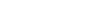, the Soft Iron effect calibration 3×3 matrix and the values of Hard Iron offset correction values b_h to be subtracted from the values recovered from the sensor output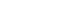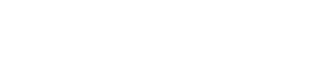There are two ways to ensure that the calibration is correct:

The first method: samples are recorded from the three axes of the sensor after calibration, and the calibration matrix is calculated again. The resulting matrix must be closer to the unitary matrix, and this means that the values are calibrated.

The second method: we use geographical directions as a reference point to know the accuracy of the calibration. For example, we use the mobile phone compass to determine the geographical north and compare that with the geographical north calculated from the magnetometer. we explained in the beginning how to calculate the geographical direction using a magnetometer.

The code below can be added to Python script after disabling the plotting part in order to find the direction in degrees:

while True:
ret = get_imu_data()
if not ret:
continue
x = ret
y = ret
z = ret

A= np.array([[1.134709 ,0.011465 ,0.069223],[0.011465 ,1.117556 ,0.057125],[0.069223 ,0.057125 ,1.710475]]) # change this array according to your calibration
B = np.array([[x],[y],[z]]) - np.array([[-1.744835] ,[-17.592499] ,[-1.965020]]) # change this array according to your calibration
Cal = np.matmul(A, B)


## A Note About Magnetometer Placement

We must keep the sensor away from any potential sources of distortion. For example, the testboard may be used during the experiment, which by itself is one of the sources of field distortion, especially for some bad quality testboards.The picture shows the best position (left) while using the breadboard, The breadboard may cause distortions in the field (right), especially the breadboard types that include metallic materials such as iron, instead of pure copper, in the composition of the internal tracks.

There is a set of design tips regarding the location of the sensor that must be taken into account when designing printed circuits and electronic devices that contain a magnetometer. I will quote the most important of them from an application note from the InvenSense company referred to it in the references.

1. The sensor should be kept away from the materials that cause distortion (Soft Iron) for a distance of at least 10 mm.
2. It is preferable to place the sensor on the edges or corners of the circuit.
3. When there is a need to add metallic materials – such as the case of having a shield – to the electronic circuit, we should choose materials with low magnetic permeability such as copper, aluminium, silver and gold. We must make a careful selection and compromisation between the material with high permeability to repel the fields from the circuit, but with a great effect on the magnetic sensor, or a material with less permeability and thus less ability to repel the electromagnetic fields, but with less effect on the sensor.
4. Keeping the sensor away from the electronic circuit lines in which currents pass through for a certain distance based on the values of the passing current.